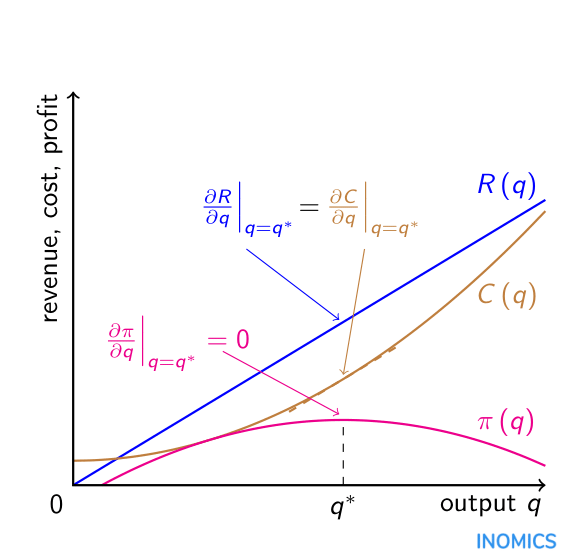# Derivatives of Functions

Derivatives of functions are computed using differential calculus. They are widely applied in economic modelling to measure the effects and rates of change in economic variables, as well as to determine maximum and minimum values of functions.

Consider the following production cost function for a good C(q) = k + aq2 where k is a fixed cost, q the number of units produced and a a variable-cost parameter. C(q) represents the total cost of production for q units of the good. When making the decision about how much of the good to produce a profit-maximizing firm will be interested in the cost of producing the last unit of the good (marginal cost) and aim to set this equal to the extra revenue from selling that unit (marginal revenue). Suppose that (total) revenue from selling this good is simply the unit price p times the quantity sold R(q) = pq. Then profit (pi) will be revenue minus cost pi(q) = R(q) C(q).

Maximum profit is found by taking the first derivative of the profit function and setting it equal to zero:
\begin{aligned} \frac{\partial\pi\left(q\right)}{\partial q}=\frac{\partial R\left(q\right)}{\partial q}-\frac{\partial C\left(q\right)}{\partial q}&=0\\ \Leftrightarrow\ \ \ \frac{\partial R\left(q\right)}{\partial q}&=\frac{\partial C\left(q\right)}{\partial q}\\ \Leftrightarrow\ \ \ p&=2aq\\ \Rightarrow\ \ \ q^{*}&=\frac{p}{2a}.\\\end{aligned}

Since the first derivative of the profit function is zero in this point, profits are neither growing nor falling. It can be verified that the point is a maximum (and not a minimum) by taking the second derivative of the profit function:

\begin{aligned} \frac{\partial^{2}\pi\left(q\right)}{\partial q^{2}}&=\frac{\partial^{2}\left(pq-\left(k+aq^{2}\right)\right)}{\partial q^{2}}\\ &=-2a,\end{aligned}

which is negative for positive a, i.e. the gradient of the profit function is decreasing in q. This means that profits are still growing at q < q* but falling for q > q* and therefore profits are at a maximum at = q*.

This example is represented in the following graph where it is shown how profits (pi) are maximized at point q = q* where the first derivative of the revenue function (marginal revenue) equals the first derivative of the cost function (marginal cost):The above example involves simple, direct, partial relationships between output quantity q and revenue/cost/profit. In most real-world situations revenue and cost will also depend on other variables, for example sales effort e and marketing expenditure m for revenue, and the price of raw materials r and wages w for cost. Insofar as these other variables interact with the production quantity, it may be necessary to calculate the total derivatives of the revenue and cost functions with respect to q in order to determine the optimal level of production,

\begin{aligned} \frac{dR\left(q,e\left(q\right),m\left(q\right)\right)}{dq}&=\frac{\partial R}{\partial q}+\frac{\partial R}{\partial e}\frac{de}{dq}+\frac{\partial R}{\partial m}\frac{dm}{dq};\\ \frac{dC\left(q,r\left(q\right),w\left(q\right)\right)}{dq}&=\frac{\partial C}{\partial q}+\frac{\partial C}{\partial r}\frac{dr}{dq}+\frac{\partial C}{\partial w}\frac{dw}{dq}.\end{aligned}

Calculating these total derivatives takes into account any indirect effects of changing the production quantity on revenue and cost that arise due to potential differences in sales effort, marketing expenditure, the price of raw materials and wages brought about by changing the production quantity.